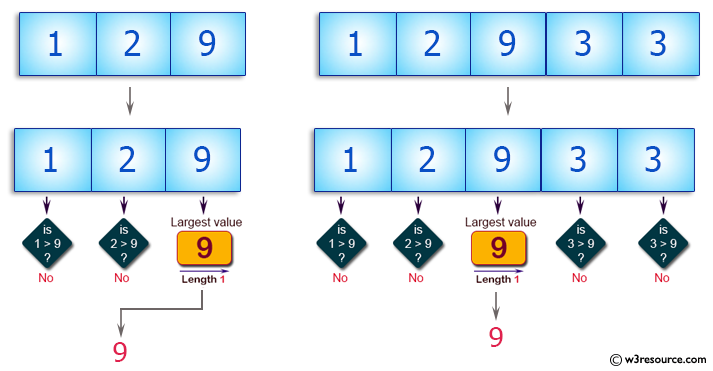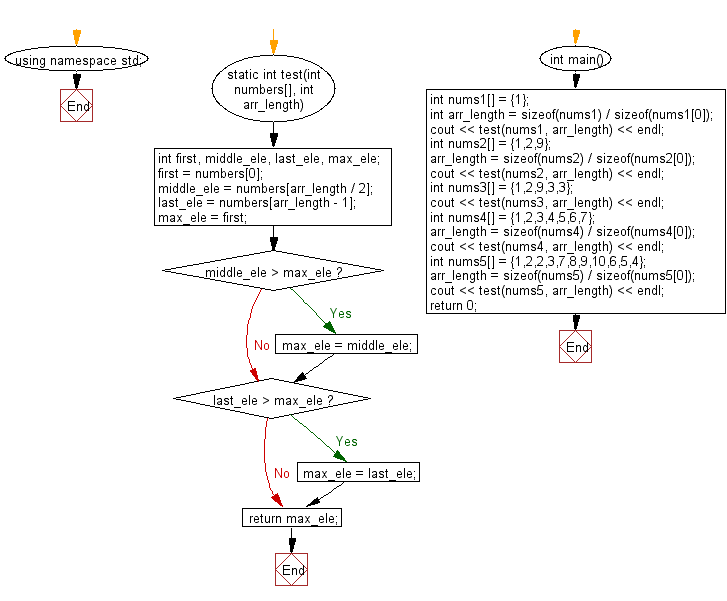﻿ C++ : Largest from first, last, middle values of an array# C++ Exercises: Find the largest value from first, last, and middle elements of a given array of integers of odd length

## C++ Basic Algorithm: Exercise-97 with Solution

Write a C++ program to find the largest value from first, last, and middle elements of a given array of integers of odd length (atleast 1).

Sample Solution:

C++ Code :

``````#include <iostream>
using namespace std;

static int test(int numbers[], int arr_length)
{
int first, middle_ele, last_ele, max_ele;

first = numbers;
middle_ele = numbers[arr_length / 2];
last_ele = numbers[arr_length - 1];
max_ele = first;

if (middle_ele > max_ele)
{
max_ele = middle_ele;
}

if (last_ele > max_ele)
{
max_ele = last_ele;
}

return max_ele;
}

int main()
{
int nums1[] = {1};
int arr_length = sizeof(nums1) / sizeof(nums1);
cout << test(nums1, arr_length) << endl;
int nums2[] = {1,2,9};
arr_length = sizeof(nums2) / sizeof(nums2);
cout << test(nums2, arr_length) << endl;
int nums3[] = {1,2,9,3,3};
cout << test(nums3, arr_length) << endl;
int nums4[] = {1,2,3,4,5,6,7};
arr_length = sizeof(nums4) / sizeof(nums4);
cout << test(nums4, arr_length) << endl;
int nums5[] = {1,2,2,3,7,8,9,10,6,5,4};
arr_length = sizeof(nums5) / sizeof(nums5);
cout << test(nums5, arr_length) << endl;
return 0;
}
``````

Sample Output:

```1
9
9
7
8
```

Pictorial Presentation:Flowchart:C++ Code Editor:

Contribute your code and comments through Disqus.

What is the difficulty level of this exercise?

﻿